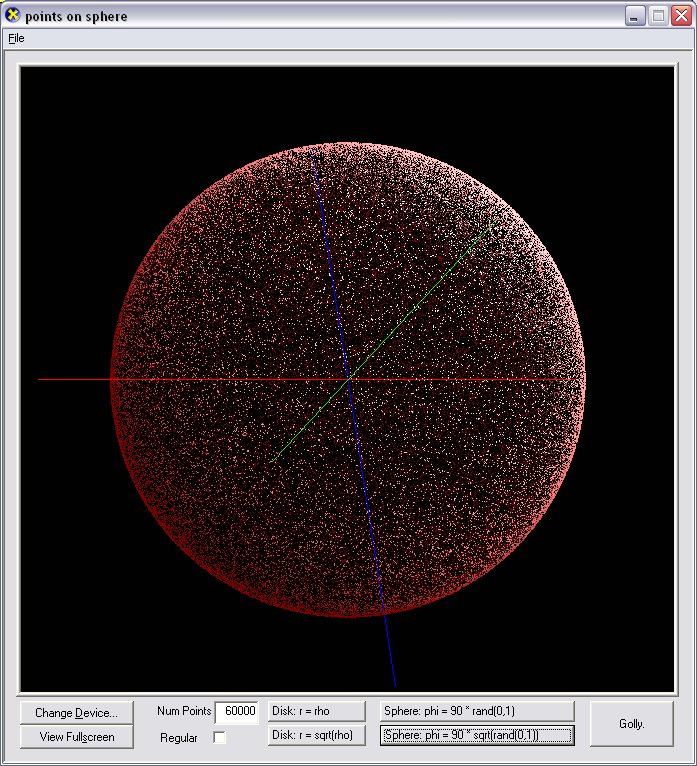## Distributing random points evenly on a sphere

Logan and i were eating indian food one night bemoaning how rusty we've become since matriculation, and i brought up "yeah, i don't even know how to go about distributing random points evenly on a sphere!" as an example, and i couldn't stop thinking about it. In the end, i've been unable to prove or even really derive the solution, but trial and error has led me to the strong belief that you just need to basically remap phi to sqrt(phi).

By 'even' i mean having the same surface density everywhere, with no Pole apparent.

This program shows the both naive and what i think is the correct method. Turns out to be exactly the same method as for distributing random points evenly over a unit disk in polar coordinates.

It is for Windows with DirectX 9.
Binary & Source included.

also of interest is distributing points regularly over a sphere, which is a bit trickier, and adressed by Paul Bourke here and by me here.

why?
Who cares ?
Well, i care, because it's interesting. But in addition to that, i realized that anyone should care who wants to frequently "choose a random direction".
- If all you need is occasionally something more or less like a random direction, like maybe your spaceship experiences an uncontrolled entry into hyperspace or seomthing, then any of these methods is fine.
But if you're choosing a random direction Frequently, then you'll want to be accurate. Examples of it mattering would include light scattering during ray tracing or (i think) radiosity, or as part of any particle system with a point or spherical emitter. That's all the practical carers i've come up with so far.

As far as efficiency, all three methods seem more or less comparable.
The naive spherical approach uses two calls to rand(), two calls to cos(), and three to sin().
The correct approach has an additional call to sqrt().
The cube approach calls rand() three times, and sqrt() once.
Actually the correct approach seems most expensive, but it's sort of a catch-22 becuase if you're only calling it every so often, then you may as well use the proper one, and if you're calling it frequently, then you better use the proper one or the unevenness in the others may become apparent.

orion x elenzil
berkeley 20040830

 naive mapping corrected mappingregular points on a plane distributed as r = rand(0, 1)regular points on a plane distributed as r = sqrt(rand(0, 1))random points on a plane distributed as r = rand(0, 1)random points on a plane distributed as r = sqrt(rand(0, 1))regular points on a (hemi)sphere distributed as phi = 90 * rand(0, 1)regular points on a (hemi)sphere distributed as phi = 90 * sqrt(rand(0, 1))random points on a (hemi)sphere distributed as phi = 90 * rand(0, 1)random points on a (hemi)sphere distributed as phi = 90 * sqrt(rand(0, 1)) the "naive/corrected" categories listed above are no longer being followed by this table, starting... now!regular points on a sphere distributed as norm(x, y, z = rand(-1, 1))regular points on a cuberandom points on a sphere distributed as norm(x, y, z = rand(-1, 1))random points on a cube